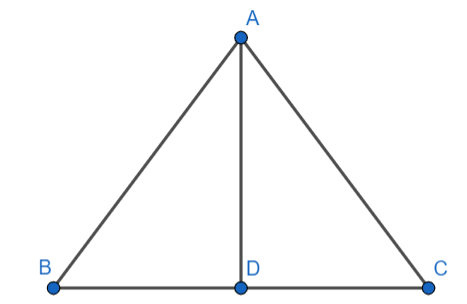QuestionAnswers

# In a triangle ABC, AD is perpendicular to BC. Prove that $A{{B}^{2}}-A{{C}^{2}}=B{{D}^{2}}-C{{D}^{2}}$.Hint: In a right-angled triangle ABC right-angled at B $A{{C}^{2}}=A{{B}^{2}}+B{{C}^{2}}$. This is known as Pythagoras theorem. Use Pythagora's theorem in triangles ABD and ACD and equate $A{{D}^{2}}$. Simplify to get the above result.

Complete step by step answer: -
Given: A triangle ABC. $\text{AD}\bot \text{BC}$.
To prove : $A{{B}^{2}}-A{{C}^{2}}=B{{D}^{2}}-C{{D}^{2}}$
Proof:
Using Pythagoras theorem in triangle ABD, we get
$\text{A}{{\text{B}}^{2}}=\text{A}{{\text{D}}^{2}}+\text{B}{{\text{D}}^{2}}$
Subtracting $\text{B}{{\text{D}}^{2}}$ from both sides we get
\begin{align} & \text{A}{{\text{B}}^{2}}-\text{B}{{\text{D}}^{2}}=\text{A}{{\text{D}}^{2}}+\text{B}{{\text{D}}^{2}}-\text{B}{{\text{D}}^{2}} \\ & \Rightarrow \text{A}{{\text{B}}^{2}}-\text{B}{{\text{D}}^{2}}=\text{A}{{\text{D}}^{2}}\text{ (i)} \\ \end{align}
Similarly, using Pythagoras theorem in triangle ACD, we get
$\text{A}{{\text{C}}^{2}}=\text{A}{{\text{D}}^{2}}+\text{C}{{\text{D}}^{2}}$
Subtracting $\text{C}{{\text{D}}^{2}}$ from both sides, we get
\begin{align} & \text{A}{{\text{C}}^{2}}-\text{C}{{\text{D}}^{2}}=\text{A}{{\text{D}}^{2}}+\text{C}{{\text{D}}^{2}}-\text{C}{{\text{D}}^{2}} \\ & \Rightarrow \text{A}{{\text{C}}^{2}}-\text{C}{{\text{D}}^{2}}=\text{A}{{\text{D}}^{2}}\text{ (ii)} \\ \end{align}
From equation (i) and equation (ii), we get
$\text{A}{{\text{B}}^{2}}-\text{B}{{\text{D}}^{2}}=\text{A}{{\text{C}}^{2}}-\text{C}{{\text{D}}^{2}}$
Adding $\text{B}{{\text{D}}^{2}}-\text{A}{{\text{C}}^{2}}$ on both sides, we get
\begin{align} & \text{A}{{\text{B}}^{2}}-\text{B}{{\text{D}}^{2}}+\text{B}{{\text{D}}^{2}}-\text{A}{{\text{C}}^{2}}=\text{A}{{\text{C}}^{2}}-\text{C}{{\text{D}}^{2}}+\text{B}{{\text{D}}^{2}}-\text{A}{{\text{C}}^{2}} \\ & \Rightarrow \text{A}{{\text{B}}^{2}}-\text{A}{{\text{C}}^{2}}=\text{B}{{\text{D}}^{2}}-\text{C}{{\text{D}}^{2}} \\ \end{align}
Hence proved.

Note: Alternatively, we can use trigonometry to prove the above statement
There are six trigonometric ratios defined on an angle of a right-angled triangle, viz sine, cosine,
tangent, cotangent, secant and cosecant.
The sine of an angle is defined as the ratio of the opposite side to the hypotenuse.
The cosine of an angle is defined as the ratio of the adjacent side to the hypotenuse.
The tangent of an angle is defined as the ratio of the opposite side to the adjacent side.
The cotangent of an angle is defined as the ratio of the adjacent side to the opposite side.
The secant of an angle is defined as the ratio of the hypotenuse to the adjacent side.
The cosecant of an angle is defined as the ratio of the hypotenuse to the adjacent side.
If $\text{A}{{\text{B}}^{2}}-\text{A}{{\text{C}}^{2}}=\text{B}{{\text{D}}^{2}}-\text{C}{{\text{D}}^{2}}$ then on dividing both sides by $\text{A}{{\text{D}}^{2}}$ we get
$\dfrac{\text{A}{{\text{B}}^{2}}-\text{A}{{\text{C}}^{2}}}{\text{A}{{\text{D}}^{2}}}=\dfrac{\text{B}{{\text{D}}^{2}}-\text{C}{{\text{D}}^{2}}}{\text{A}{{\text{D}}^{2}}}$
We know that $\dfrac{a-b}{c}=\dfrac{a}{c}-\dfrac{b}{c}$.
Using the above identity, we get
${{\left( \dfrac{\text{AB}}{\text{AD}} \right)}^{2}}-{{\left( \dfrac{\text{AC}}{\text{AD}} \right)}^{2}}={{\left( \dfrac{\text{BD}}{\text{AD}} \right)}^{2}}-{{\left( \dfrac{\text{CD}}{\text{AD}} \right)}^{2}}$
In triangle ABD we have
$\csc \text{B=}\dfrac{\text{AB}}{\text{AD}}$ and $\cot \text{B=}\dfrac{\text{BD}}{\text{AD}}$
In triangle ACD we have
$\csc \text{C=}\dfrac{\text{AC}}{\text{AD}}$ and $\cot \text{C=}\dfrac{\text{CD}}{\text{AD}}$
Substituting these values, we get
${{\csc }^{2}}\text{B-}{{\csc }^{2}}\text{C=}{{\cot }^{2}}\text{B-}{{\cot }^{2}}\text{C}$
Adding ${{\csc }^{2}}\text{C-co}{{\text{t}}^{2}}\text{B}$ on both sides, we get
\begin{align} & {{\csc }^{2}}\text{B-}{{\csc }^{2}}\text{C+}{{\csc }^{2}}\text{C-co}{{\text{t}}^{2}}\text{B=}{{\cot }^{2}}\text{B-}{{\cot }^{2}}\text{C+}{{\csc }^{2}}\text{C-co}{{\text{t}}^{2}}\text{B} \\ & {{\csc }^{2}}\text{B}-{{\cot }^{2}}\text{B=}{{\csc }^{2}}\text{C-}{{\cot }^{2}}\text{C} \\ \end{align}
We know that ${{\csc }^{2}}x-{{\cot }^{2}}x=1$
Using we have $1=1$
Which is true.
Hence $\text{A}{{\text{B}}^{2}}-\text{A}{{\text{C}}^{2}}=\text{B}{{\text{D}}^{2}}-\text{C}{{\text{D}}^{2}}$
Hence proved.
Right Angled Triangle ConstructionsThe Difference Between an Animal that is A Regulator and One that is A ConformerWhere There is a Will There is a Way EssayCBSE Class 10 Maths Chapter 6 - Triangles FormulaLaughter is a Best Medicine EssayCBSE Class 7 Maths Chapter 6 - Triangle and Its Properties FormulasGroup Theory in MathematicsCBSE Class 10 Maths Chapter 8 - Introduction to Trigonometry FormulaIMO Maths Olympiad Sample Question Paper for Class 6 to 10How To Make A Buzzer?Important Questions for CBSE Class 10 Maths Chapter 8 - Introduction to TrigonometryImportant Questions for CBSE Class 10 Maths Chapter 12 - Areas Related to CirclesImportant Questions for CBSE Class 10 Maths Chapter 6 - TrianglesImportant Questions for CBSE Class 8 English Honeydew Chapter 7 - A Visit to CambridgeImportant Questions for CBSE Class 6 English A Pact with The Sun Chapter 10 - A Strange Wrestling MatchImportant Questions for CBSE Class 10 Maths Chapter 10 - CirclesImportant Questions for CBSE Class 11 Physics Chapter 4 - Motion in a PlaneImportant Questions for CBSE Class 10 Maths, Chapter wise Questions with AnswersNCERT Books for Class 10 MathsCBSE Class 10 Hindi A Question Paper 2020Hindi A Class 10 CBSE Question Paper 2009Hindi A Class 10 CBSE Question Paper 2015Hindi A Class 10 CBSE Question Paper 2008Hindi A Class 10 CBSE Question Paper 2014Hindi A Class 10 CBSE Question Paper 2016Hindi A Class 10 CBSE Question Paper 2012Hindi A Class 10 CBSE Question Paper 2010Hindi A Class 10 CBSE Question Paper 2007Hindi A Class 10 CBSE Question Paper 2013RS Aggarwal Class 9 Solutions Chapter-9 Congruence of Triangles and Inequalities in a TriangleNCERT Solutions for Class 10 Maths Chapter 6 TrianglesNCERT Solutions for Class 10 Maths Chapter 8 Introduction to Trigonometry in HindiRS Aggarwal Class 10 Solutions - TrianglesNCERT Solutions for Class 10 Maths Chapter 12 Areas Related to Circles in HindiRD Sharma Solutions for Class 9 Maths Chapter 10 - Congruent TrianglesRD Sharma Solutions for Class 10 Maths Chapter 4 - TrianglesNCERT Solutions for Class 10 English First Flight Chapter 1 - A Letter to GodNCERT Solutions for Class 10 Maths Chapter 6 Triangles in HindiNCERT Solutions for Class 7 Maths Chapter 6 The Triangle and Its Properties In Hindi